# 排序——快速排序# 快速排序

## 基本思想

• 任取一个元素 (如第一个) 为中心
• 所有比它的元素一律前放，比它的元素一律后放，形成左右两个子表
• 对各子表重新选择中心元素并依此规则调整，直到每个子表的元素只剩一个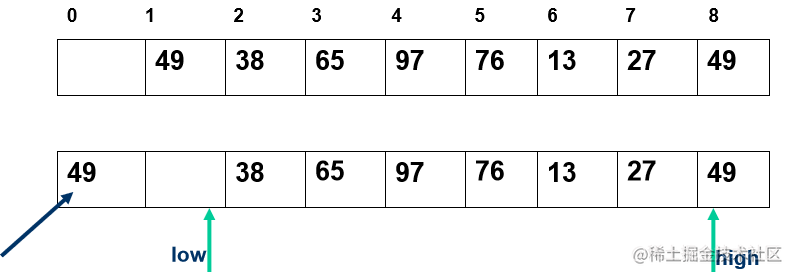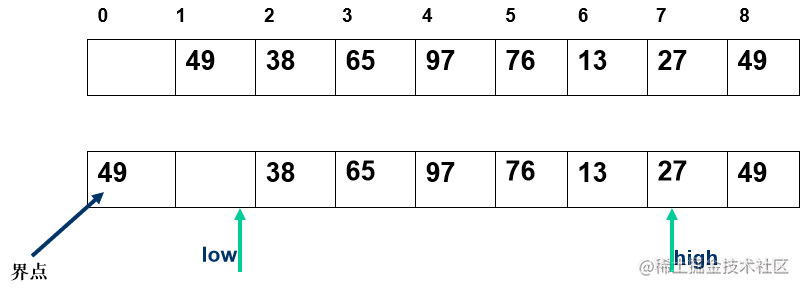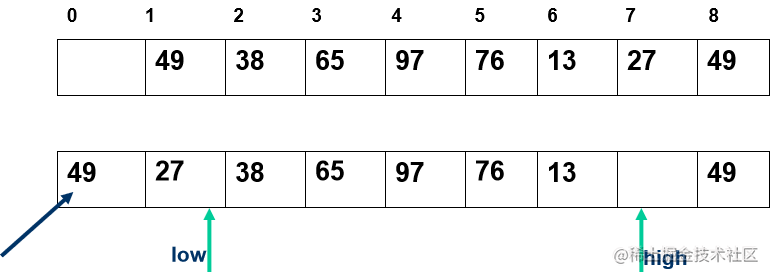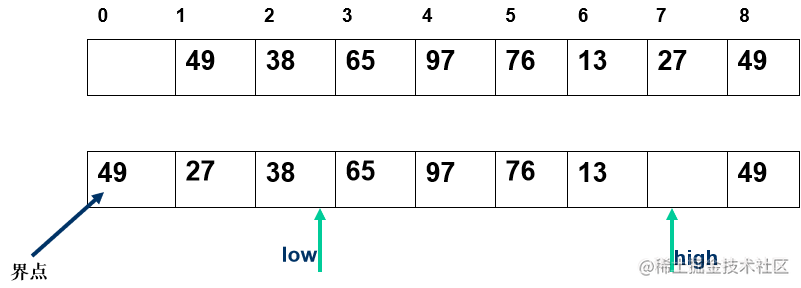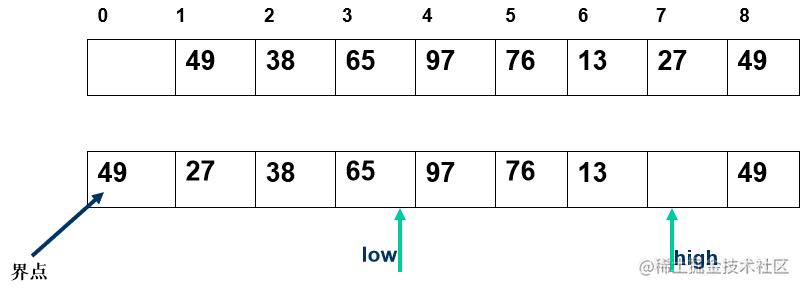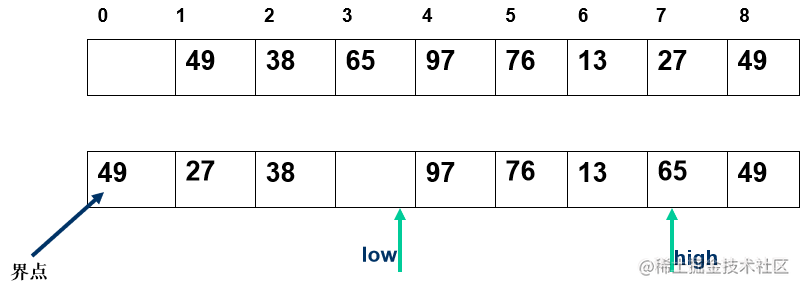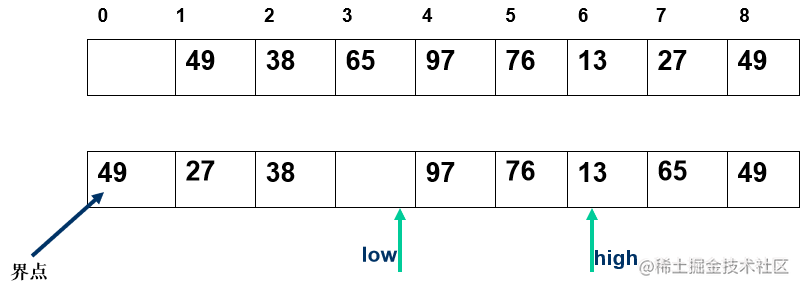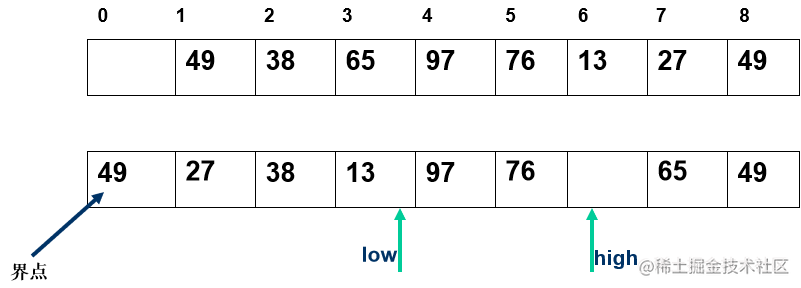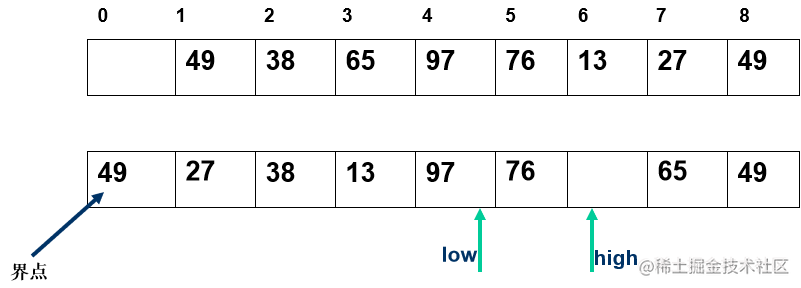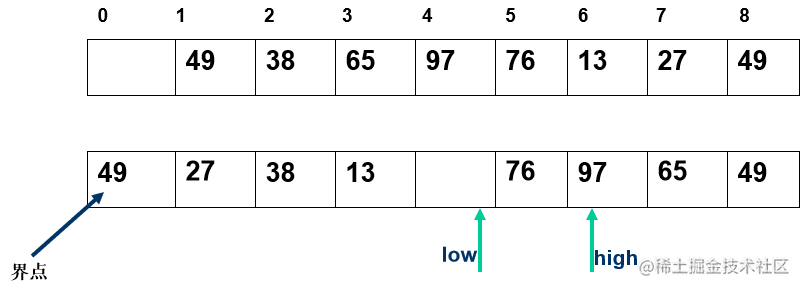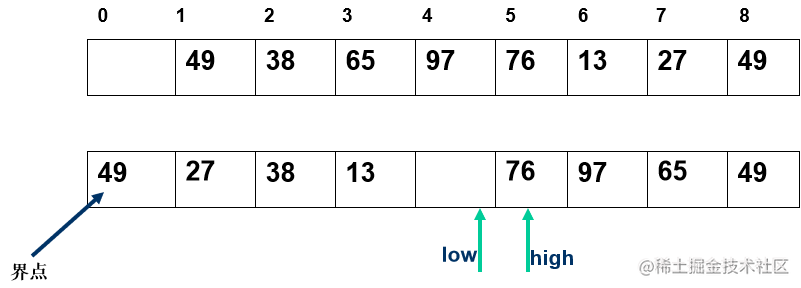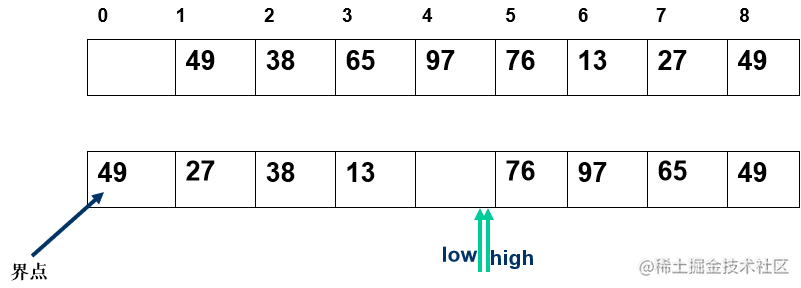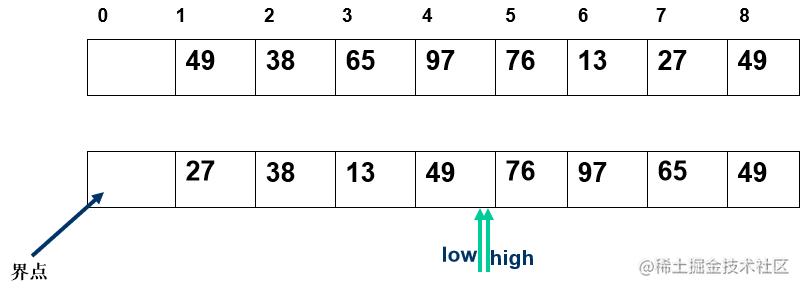## 算法实现

``````int Partition(SqList &L, int low, int high){
L.r = L.r[low];
pivotkey = L.r[low].key;
while(low < high){
while(low < high && L.r[high].key >= pivotkey)
--high;  // 从右向左搜索
L.r[low] = L.r[high];
while(low < high && L.r[low].key <= pivotkey)
++low;  // 从左向右搜索
L.r[high] = L.r[low];
}
L.r[low] = L.r;
return low;
}

void QSort(SqList &L, int low, int high){
// 对记录序列L[low..high]进行快速排序
if(low < high){
// 长度大于1
pivotkey = Partition(L, low, high);  // 对 L[low..high] 进行一次划分
QSort(L, low, pivotloc-1);  // 对低子表递归排序，pivotloc是枢轴位置
QSort(L, pivotloc+1, high); // 对高子表递归排序
}
}

// 第一次调用函数 Qsort 时，待排序记录序列的上、下界分别为 1 和 L.length。
void QuickSort( SqList & L) {
// 对顺序表进行快速排序
QSort(L.r, 1, L.length);
}

## 算法分析

• 时间复杂度：O(n^2)
• 最好： O(n log2n )
• 最坏：O(n^2)
• 平均：O(nlogn)
• 空间复杂度：O(n)
• O（log2n）—递归要用到栈空间
• 最坏情况下，递归树的高度为O(n)
• 稳定性：不稳定